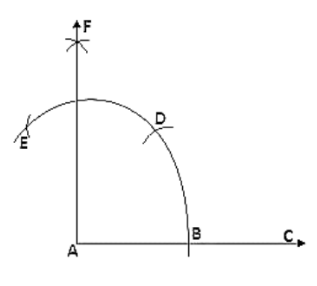# Using rulers and compasses only, draw a right angle.

Question:

Using rulers and compasses only, draw a right angle.

Solution:

Steps of construction:

1. Draw a line segment AB.

2. Keeping A as the center and any radius draw an arc which intersects AB at C.

3. Keeping C as center and the same radius draw an arc which intersects the previous arc at D.

4. Keeping D as the center and same radius draw an arc which intersects arc in (2) at E.

5. Keeping E and D as center and radius more than half of ED draw arcs which intersect each other at F.

6. Join FA.

Therefore ∠FAB = 90°